# Position, Distance and Displacement

Welcome to class!

In today’s class, we will be talking about position, distance and displacement. Enjoy the class!

###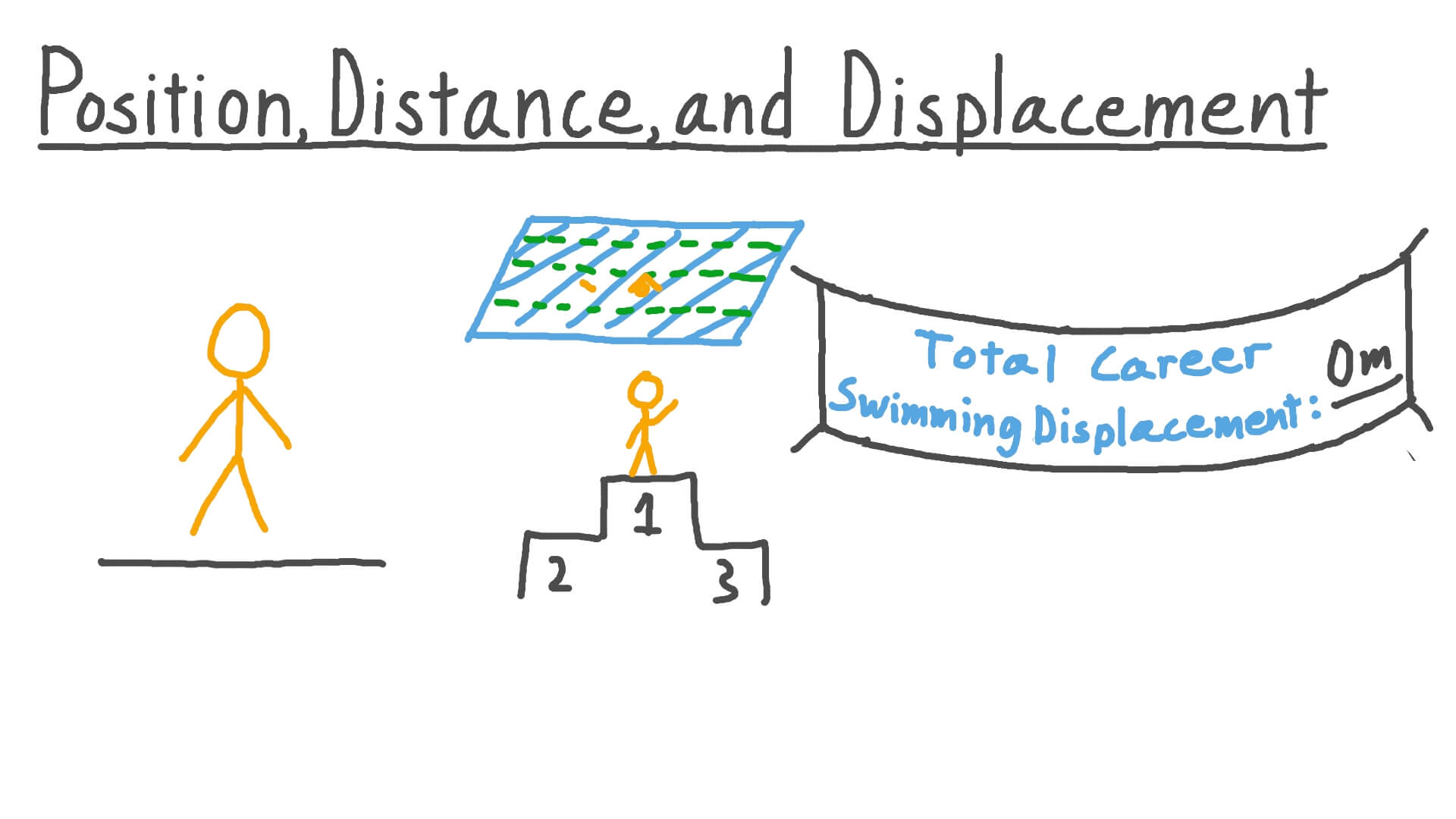#### POSITION

Position is a point in space, is determined by its distance and direction from other points. The statement of position is accomplished by means of a frame of reference or a point of reference, which is called the Origin. Position is a location of a point using rectangular coordinates, x and y axes. When writing the coordinates of points, x is written first followed by y.

WORKED EXAMPLE

(1) Point A (2, -3) has the value of x as 2 and that of y as – 3. Represent this on the Cartesian plane.

SOLUTION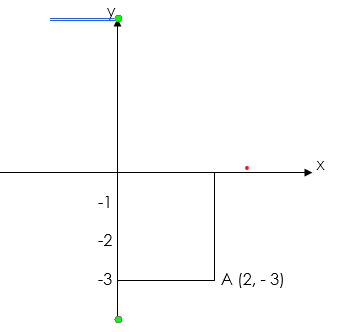NOTE:

The x – coordinate is also known as abscissa while the y – coordinate is known as ordinate.

#### DISTANCE

Distance is the product of average speed and time.

Distance = average speed x time

Distance is also defined as the length of space covered by an object when it changes position from one point to another. Note that the direction is not specified hence distance is a scalar quantity. The S.I unit is the metre (m).

#### DISPLACEMENT

Displacement is the distance travelled in a constant direction.

Displacement average velocity x time

Displacement is also defined as the distance a body moves in a specified direction. It is, therefore, a vector quantity.

It is denoted by the letter ‘s’ and has both magnitude and direction. The SI unit for displacement is the metre (m).##### WORKED EXAMPLES

(1) A body moving with a constant velocity along a straight line ABC takes 45 minutes to go from A to B and 10 minutes from B to C. if Ac is 3km find AB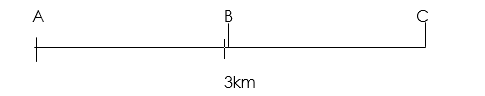SOLUTION

Average Velocity:But displacement s = average velocity, v x time, t

Therefore: Displacement AB = 3/55 x 45 = 2.45 km.

(2) A man walks 1 km due East and then 1 km due North. Find the displacement.

SOLUTIONR2 = 12 + 12

R2 = 2

R =

tan  =  = 1

= 45°

Therefore the displacement R =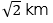N 45° E

(3) Calculate the distance in metres covered by a body moving with a uniform speed of 180 km/h in 30 seconds.

SOLUTION

Given parameters

Time = 30 seconds

Uniform speed = 180km/r

Converting from km/hr to m/sTherefore

Distance covered= Speed x time

=50 x 30

=1500 m

(4) A body is uniformly accelerated from rest to a final velocity of 100m/s in 10 seconds. Calculate the distance covered.

SOLUTION

Given parameters

Velocity (u) = 100m/s

Time = 10seconds

Note: Velocity is given so we apply the equation of motion

Therefore (a = 10m/s2)

S=ut+ ½ at2

Substituting:

=0×10+ ½ ×10×102

=  = 500 m

##### Distance between two coordinates pointsLet P P(x1, y1) and Q(x2, y2) be any two points. The distance between P and Q is found by completing the right-angled triangle PQR with the right angle at R by applying Pythagoras Theorem.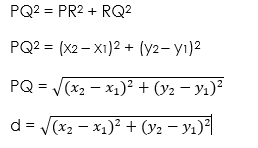##### WORKED EXAMPLES

(1) Find the distance between the two coordinates A(3, 2) and (6, 4)

SOLUTION

The distance (d) between two points (x2 – x1) and (y2 – y1) is defined by:(2) Find the value of x, given the following coordinates A(1, -2) and B(x, -5) with a distance of 5.

The distance (d) between two points (x2 – x1) and (y2 – y1) is defined by:

Given parameters:

d = 5

(x1 – y1) = (1, -2) and (x2 – y2) = (x, -5)

Substituting: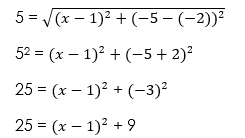Collect like terms

25 – 9 =

16 =

Take square root of both sides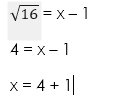x = 5

Therefore x = 5

A(1, -2) and B(5, -5)

In our next class, we will be talking about Physical Quantities.  We hope you enjoyed the class.

Should you have any further question, feel free to ask in the comment section below and trust us to respond as soon as possible.

How Can We Make ClassNotesNG Better - CLICK to Tell Us💃

### 28 thoughts on “Position, Distance and Displacement”

1.I enjoyed today’s lesson

1.I love today class

2.I love today’s class

3.Determine the distance between S(3,4,-5)and T(2,1,0)

4.what is the distance between point A (9,6) and point B (5,3)

5.Great job. I so much appreciate you. This note was really useful and helpful.

6.Thanks for this, its a great resource

1.Yes this is so helpful, it helped me to study

7.IT is very good and

8.Wow interesting
Keep up the work

9.I enjoyed the class well. Keep it up

10.Thank you so much,l love this lesson

11.Thank you so much l love this lesson

12.I like the class it is fantastic and is understandable to really nice

13.This has helped me a lot😊👍

1.I agree

14.HOW CAN I JOIN THE CLASSES

15.This class is damn great

16.I can I join

17.Today’s class was awesome

18.how can i join the classes plss

19.Wow, really comprehensive

20.On distance between two coordinates points is there not an explanation where graph is needed

21.U love today class but ,I don’t understand the worked one number 4

22.Thanks you so much Sir

Don`t copy text!